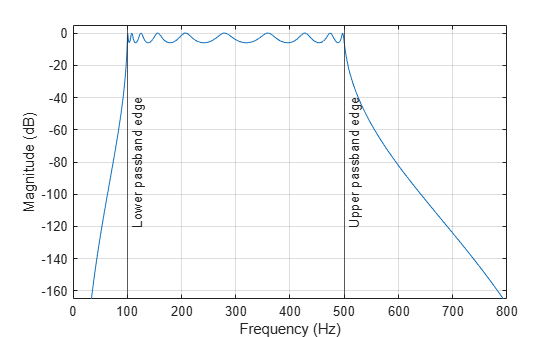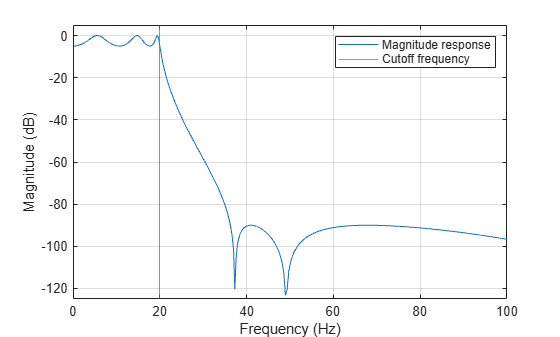# bilinear

Bilinear transformation method for analog-to-digital filter conversion

## Syntax

``[zd,pd,kd] = bilinear(z,p,k,fs)``
``[numd,dend] = bilinear(num,den,fs)``
``[Ad,Bd,Cd,Dd] = bilinear(A,B,C,D,fs)``
``[___] = bilinear(___,fp)``

## Description

````[zd,pd,kd] = bilinear(z,p,k,fs)` converts the s-domain transfer function in pole-zero form specified by `z`, `p`, `k` and sample rate `fs` to a discrete equivalent.```
````[numd,dend] = bilinear(num,den,fs)` converts the s-domain transfer function specified by numerator `num` and denominator `den` to a discrete equivalent.```

example

````[Ad,Bd,Cd,Dd] = bilinear(A,B,C,D,fs)` converts the continuous-time state-space system in matrices `A`, `B`, `C`, and `D` to a discrete-time system.```

example

````[___] = bilinear(___,fp)`uses parameter `fp` as "match" frequency to specify prewarping.```

## Examples

collapse all

Design the prototype for a 10th-order Chebyshev type I bandpass filter with 3 dB of ripple in the passband. Convert it to state-space form.

```[z,p,k] = cheb1ap(10,3); [A,B,C,D] = zp2ss(z,p,k); ```

Create an analog filter with sample rate ${\mathit{f}}_{\mathit{s}}=2\text{\hspace{0.17em}}\mathrm{kHz}$, prewarped band edges ${\mathit{u}}_{1}\text{\hspace{0.17em}}$and ${\mathit{u}}_{2}$ in rad/s, bandwidth ${\mathit{B}}_{\mathit{w}}={\mathit{u}}_{2}-{\mathit{u}}_{1}$ and center frequency ${\mathit{W}}_{\mathit{o}}=\sqrt{{\mathit{u}}_{1}{\mathit{u}}_{2}}$ for use with `lp2bp`. Specify the passband edge frequencies as 100 Hz and 500 Hz.

```Fs = 2e3; u1 = 2*Fs*tan(100*(2*pi/Fs)/2); u2 = 2*Fs*tan(500*(2*pi/Fs)/2); Bw = u2 - u1; Wo = sqrt(u1*u2); [At,Bt,Ct,Dt] = lp2bp(A,B,C,D,Wo,Bw); [b,a] = ss2tf(At,Bt,Ct,Dt); ```

Calculate the frequency response of the analog filter using `freqs`. Plot the magnitude response and the prewarped frequency band edges.

```[h,w] = freqs(b,a); plot(w,mag2db(abs(h))) hold on ylim([-165 5]) [U1,U2] = meshgrid([u1 u2],ylim); plot(U1,U2) legend('Magnitude response','Lower Passband Edge','Upper Passband Edge') hold off xlabel('Angular Frequency (rad/s)') ylabel('Magnitude (dB)') grid```Use `bilinear` to create a digital bandpass filter with sample rate ${\mathit{f}}_{\mathit{s}}$ and lower band edge 100 Hz. Convert the digital filter from state-space form to transfer function form using `ss2tf`.

```[Ad,Bd,Cd,Dd] = bilinear(At,Bt,Ct,Dt,Fs); [bz,az] = ss2tf(Ad,Bd,Cd,Dd);```

Use `fvtool` to plot the magnitude response of the digital filter.

`fvtool(bz,az,'Fs',Fs)`Design a 6th-order elliptic analog lowpass filter with 3 dB of ripple in the passband and a stopband 90 dB down. Set cutoff frequency ${\mathit{f}}_{\mathit{c}}=20\text{\hspace{0.17em}}\mathrm{Hz}$ and sample rate ${\mathit{f}}_{\mathit{s}}=200\text{\hspace{0.17em}}\mathrm{Hz}$.

```clear Fc = 20; Fs = 200; [z,p,k] = ellip(6,3,90,2*pi*Fc,'s'); [num,den] = zp2tf(z,p,k);```

Calculate the magnitude response of the analog elliptic filter. Visualize the analog filter.

```[h,w] = freqs(num,den); plot(w/(2*pi),mag2db(abs(h))) hold on xlim([0 50]) [l1,l2] = meshgrid(Fc,[-120 0]); plot(l1,l2) grid legend('Magnitude response','Passband Edge') xlabel('Frequency (Hz)') ylabel('Magnitude (dB)')```Use `bilinear` to transform it to a discrete-time IIR filter. Set the match frequency as ${\mathit{f}}_{\mathit{p}}=20\text{\hspace{0.17em}}\mathrm{Hz}$.

`[numd,dend] = bilinear(num,den,Fs,20);`

Visualize the filter using `fvtool`.

`fvtool(numd,dend,'Fs',Fs) `## Input Arguments

collapse all

Zeros of the s-domain transfer function, specified as a column vector.

Poles of the s-domain transfer function, specified as a column vector.

Gain of the s-domain transfer function, specified as a scalar.

Sample rate, specified as a positive scalar.

Numerator coefficients of the analog transfer function, specified as a row vector.

Denominator coefficients of the analog transfer function, specified as a row vector.

State matrix in the s-domain, specified as a matrix. If the system has p inputs and q outputs and is described by n state variables, then `A` is n-by-n.

Data Types: `single` | `double`

Input-to-state matrix in the s-domain, specified as a matrix. If the system has p inputs and q outputs and is described by n state variables, then `B` is n-by-p.

Data Types: `single` | `double`

State-to-output matrix in the s-domain, specified as a matrix. If the system has p inputs and q outputs and is described by n state variables, then `C` is q-by-n.

Data Types: `single` | `double`

Feedthrough matrix in the s-domain, specified as a matrix. If the system has p inputs and q outputs and is described by n state variables, then `D` is q-by-p.

Data Types: `single` | `double`

Match frequency, specified as a positive scalar.

## Output Arguments

collapse all

Zeros of the z-domain transfer function, specified as a column vector.

Poles of the z-domain transfer function, specified as a column vector.

Gain of the z-domain transfer function, specified as a scalar.

Numerator coefficients of the digital transfer function, specified as a row vector.

Denominator coefficients of the digital transfer function, specified as a row vector.

State matrix in the z-domain, returned as a matrix. If the system is described by n state variables, then `Ad` is n-by-n.

Data Types: `single` | `double`

Input-to-state matrix in the z-domain, returned as a matrix. If the system is described by n state variables, then `Bd` is n-by-1.

Data Types: `single` | `double`

State-to-output matrix in the z-domain, returned as a matrix. If the system has q outputs and is described by n state variables, then `Cd` is q-by-n.

Data Types: `single` | `double`

Feedthrough matrix in the z-domain, returned as a matrix. If the system has q outputs, then `Dd` is q-by-1.

Data Types: `single` | `double`

## Diagnostics

`bilinear` requires that the numerator order be no greater than the denominator order. If this is not the case, `bilinear` displays

```Numerator cannot be higher order than denominator. ```

For `bilinear` to distinguish between the zero-pole-gain and transfer function linear system formats, the first two input parameters must be vectors with the same orientation in these cases. If this is not the case, `bilinear` displays

```First two arguments must have the same orientation. ```

## Algorithms

collapse all

The bilinear transformation is a mathematical mapping of variables. In digital filtering, it is a standard method of mapping the s or analog plane into the z or digital plane. It transforms analog filters, designed using classical filter design techniques, into their discrete equivalents.

The bilinear transformation maps the s-plane into the z-plane by

`${H\left(z\right)=H\left(s\right)|}_{s=2{f}_{s}\frac{z-1}{z+1}}.$`

This transformation maps the jΩ axis (from Ω = –∞ to +∞) repeatedly around the unit circle (ejw, from ω = –π to π) by

`$\omega =2{\mathrm{tan}}^{-1}\left(\frac{\Omega }{2{f}_{s}}\right).$`

`bilinear` can accept an optional parameter `Fp` that specifies prewarping. `fp`, in hertz, indicates a “match” frequency, that is, a frequency for which the frequency responses before and after mapping match exactly. In prewarped mode, the bilinear transformation maps the s-plane into the z-plane with

`${H\left(z\right)=H\left(s\right)|}_{s=\frac{2\pi {f}_{p}}{\mathrm{tan}\left(\pi \frac{{f}_{p}}{{f}_{s}}\right)}\frac{z-1}{z+1}}.$`

With the prewarping option, `bilinear` maps the jΩ axis (from Ω = –∞ to +∞) repeatedly around the unit circle (e, from ω = –π to π) by

`$\omega =2{\mathrm{tan}}^{-1}\left(\frac{\Omega \mathrm{tan}\left(\pi \frac{{f}_{p}}{{f}_{s}}\right)}{2\pi {f}_{p}}\right).$`

In prewarped mode, `bilinear` matches the frequency 2πfp (in radians per second) in the s-plane to the normalized frequency 2πfp/fs (in radians per second) in the z-plane.

The `bilinear` function works with three different linear system representations: zero-pole-gain, transfer function, and state-space form.

`bilinear` uses one of two algorithms depending on the format of the input linear system you supply. One algorithm works on the zero-pole-gain format and the other on the state-space format. For transfer function representations, `bilinear` converts to state-space form, performs the transformation, and converts the resulting state-space system back to transfer function form.

### Zero-Pole-Gain Algorithm

For a system in zero-pole-gain form, `bilinear` performs four steps:

1. If `fp` is present, it prewarps:

```fp = 2*pi*fp; fs = fp/tan(fp/fs/2) ```

otherwise, `fs = 2*fs`.

2. It strips any zeros at ±∞ using

```z = z(finite(z)); ```
3. It transforms the zeros, poles, and gain using

```pd = (1+p/fs)./(1-p/fs); % Do bilinear transformation zd = (1+z/fs)./(1-z/fs); kd = real(k*prod(fs-z)./prod(fs-p)); ```
4. It adds extra zeros at -1 so the resulting system has equivalent numerator and denominator order.

### State-Space Algorithm

An analog system in state space form is given by

`$\begin{array}{l}\stackrel{˙}{x}=Ax+Bu\\ y=Cx+Du\end{array}$`

. This system is converted to the discrete form using state-space equations as follows:

To convert an analog system in state-space form, `bilinear` performs two steps:

1. If `fp` is present, let

`$\lambda =\frac{\pi {f}_{p}}{\mathrm{tan}\left(\pi {f}_{p}/{f}_{s}\right)}.$`

If `fp` is not present, let λ=fs.

2. Compute `Ad`, `Bd`, `Cd`, and `Dd` in terms of `A`, `B`, `C`, and `D` using

`$\begin{array}{l}{A}_{d}={\left(}^{I}\left(I+A\frac{1}{2\lambda }\right),\\ {B}_{d}=\frac{1}{\sqrt{\lambda }}{\left(}^{I}B,\\ {C}_{d}=\frac{1}{\sqrt{\lambda }}C{\left(}^{I},\\ {D}_{d}=\frac{1}{2\lambda }C{\left(}^{I}B+D.\end{array}$`

### Transfer Function

For a system in transfer function form, `bilinear` converts an s-domain transfer function given by `num` and `den` to a discrete equivalent. Row vectors `num` and `den` specify the coefficients of the numerator and denominator, respectively, in descending powers of s. Let B(s) be the numerator polynomial and A(s) be the denominator polynomial. The transfer function is:

`$\frac{B\left(s\right)}{A\left(s\right)}=\frac{B\left(1\right){s}^{n}+\cdots +B\left(n\right)s+B\left(n+1\right)}{A\left(1\right){s}^{m}+\cdots +A\left(m\right)s+A\left(m+1\right)}$`

`fs` is the sample rate in hertz. `bilinear` returns the discrete equivalent in row vectors `numd` and `dend` in descending powers of z (ascending powers of z–1). `fp` is the optional match frequency, in hertz, for prewarping.

 Oppenheim, Alan V., Ronald W. Schafer, and John R. Buck. Discrete-Time Signal Processing. Upper Saddle River, NJ: Prentice Hall, 1999.

 Parks, Thomas W., and C. Sidney Burrus. Digital Filter Design. New York: John Wiley & Sons, 1987.